Successfully reported this slideshow.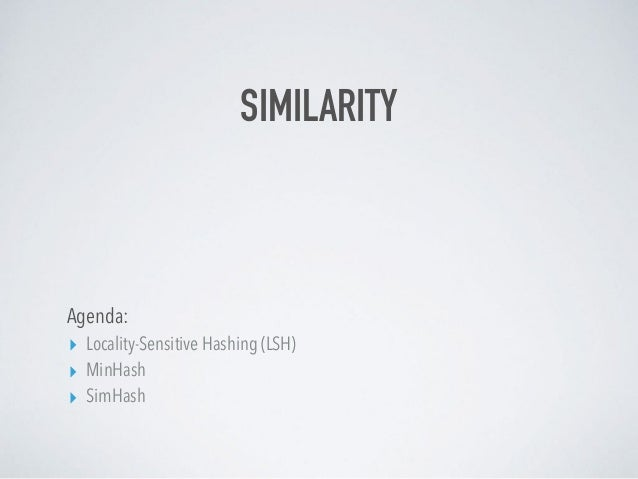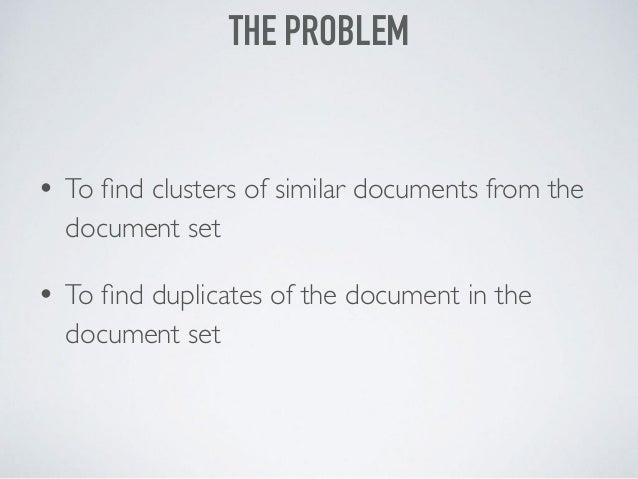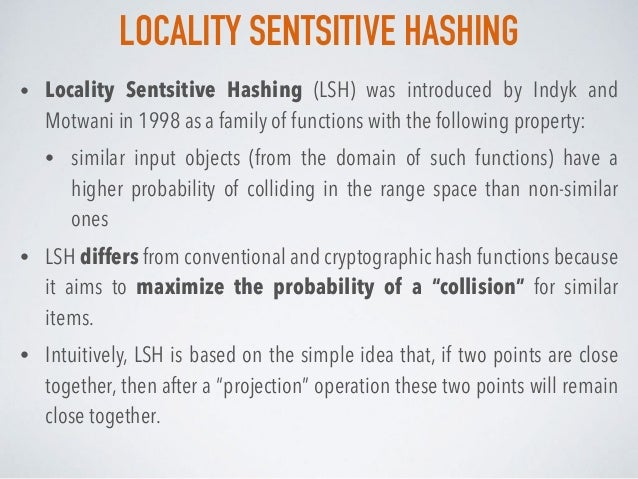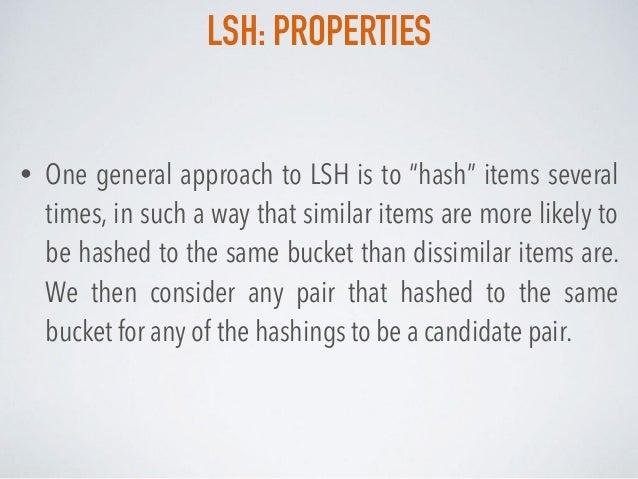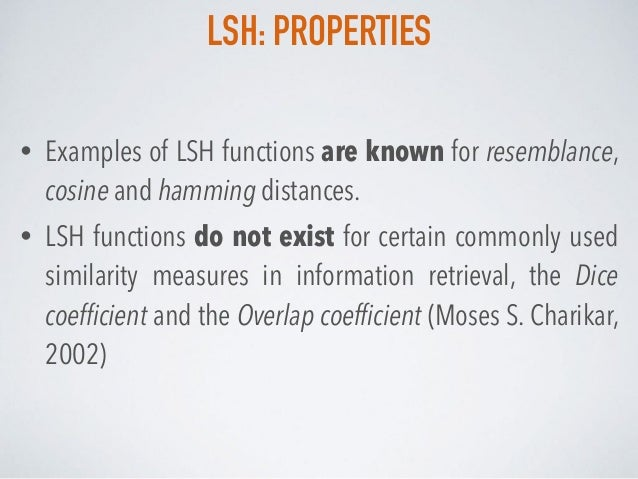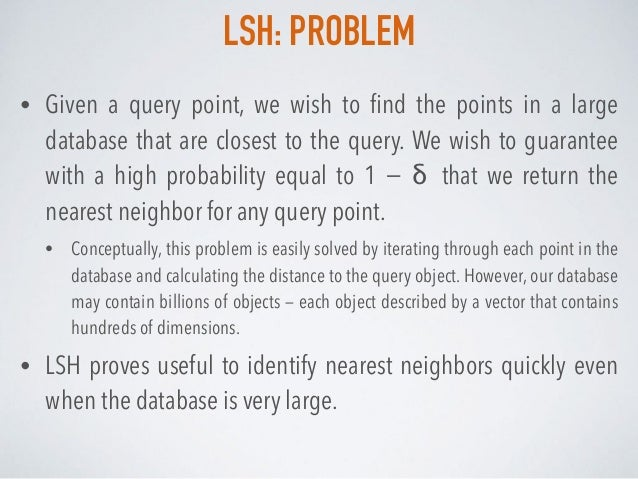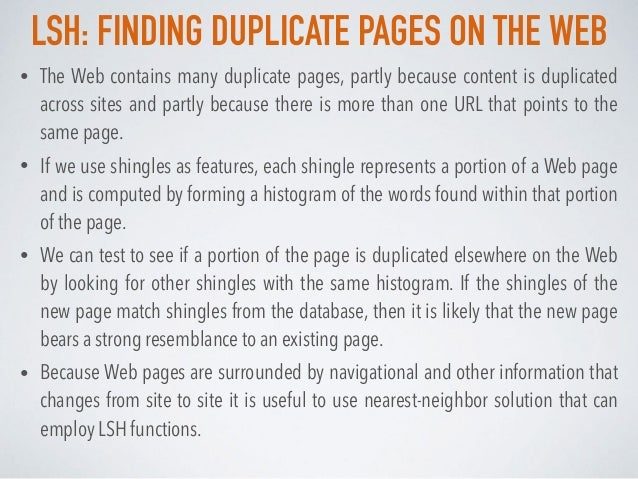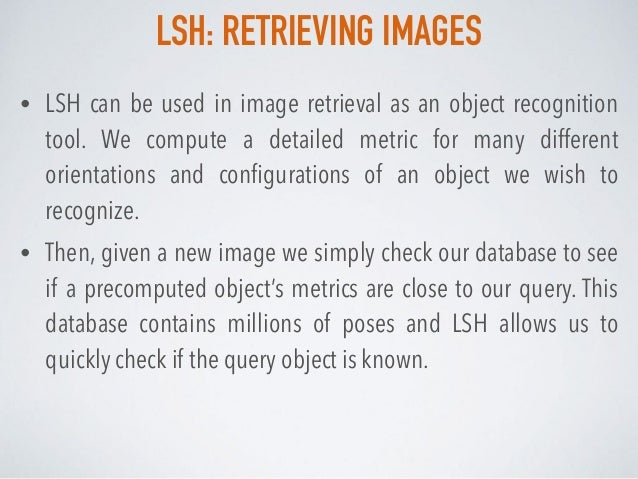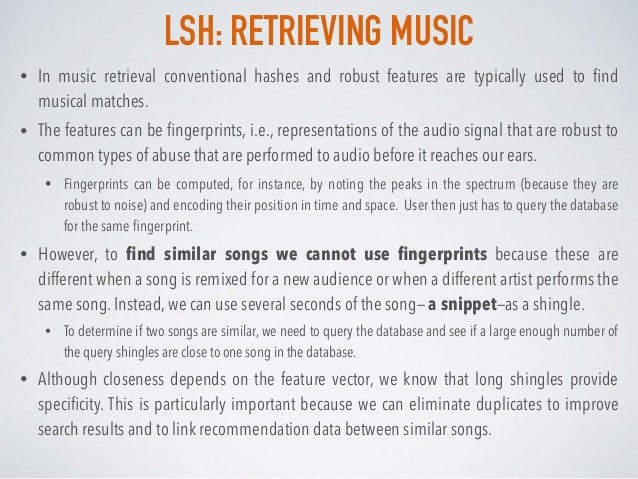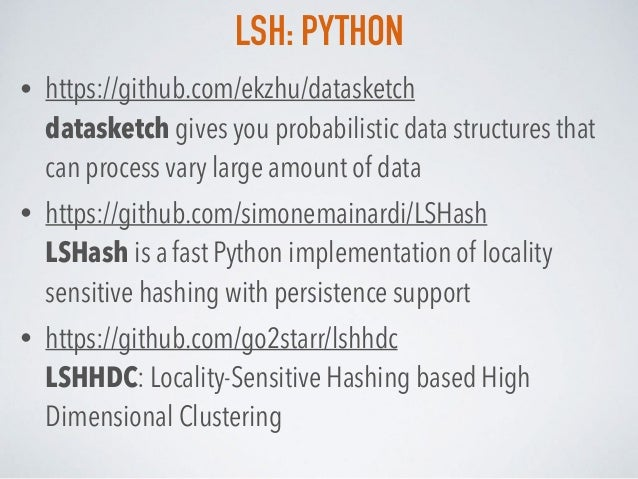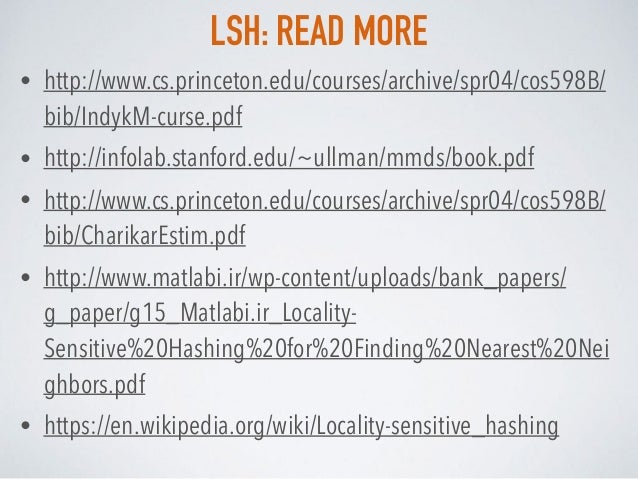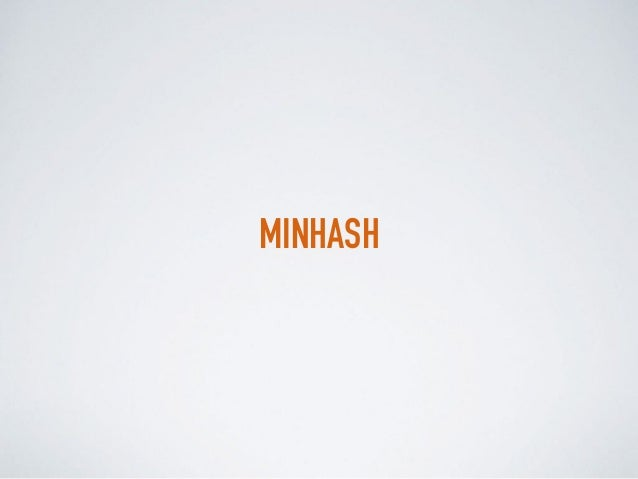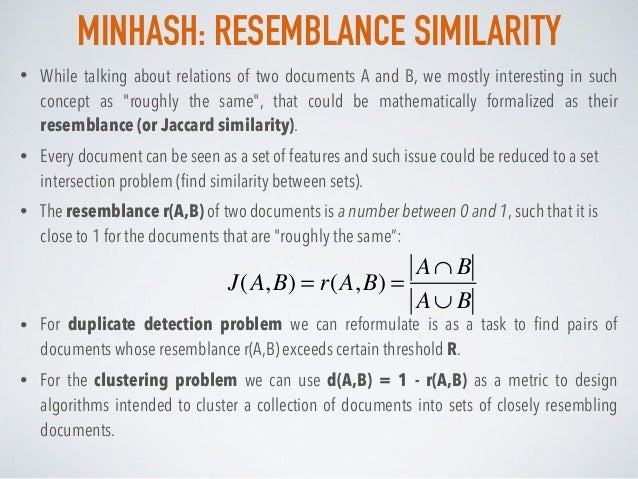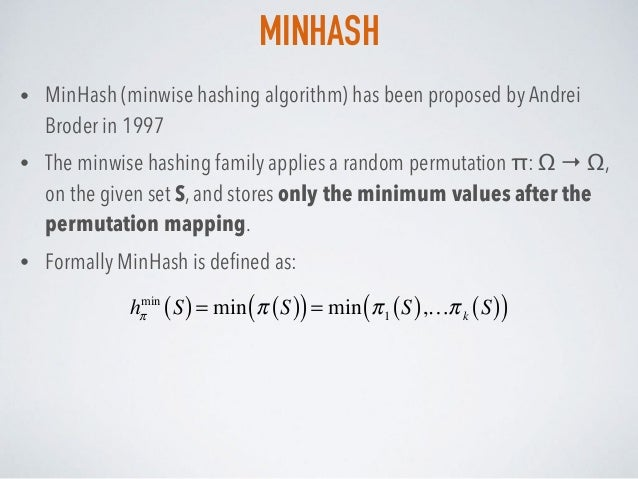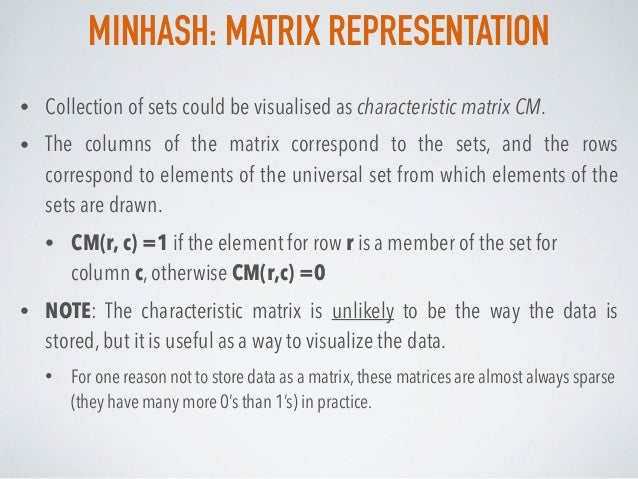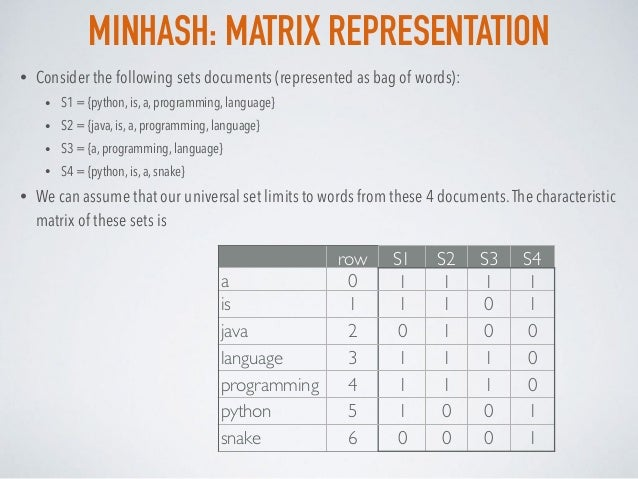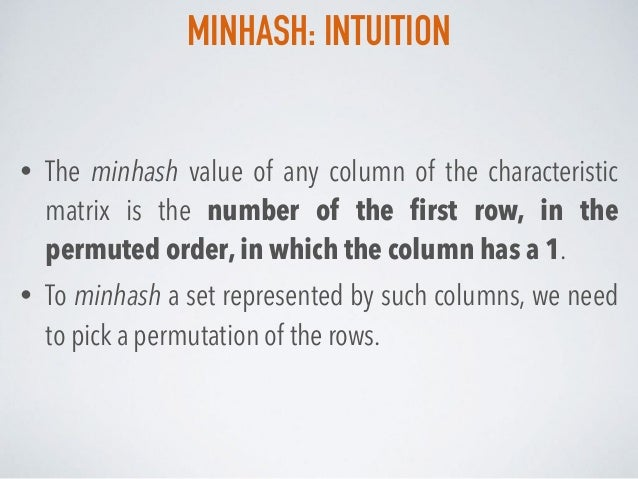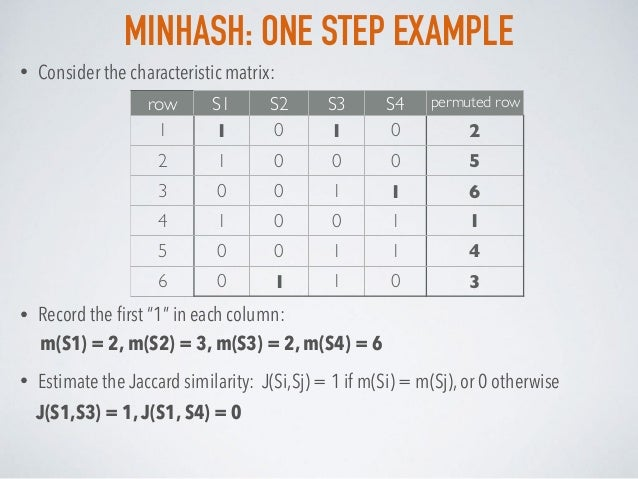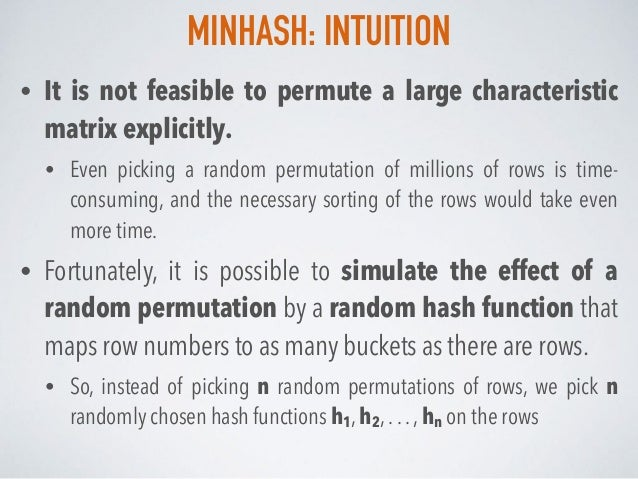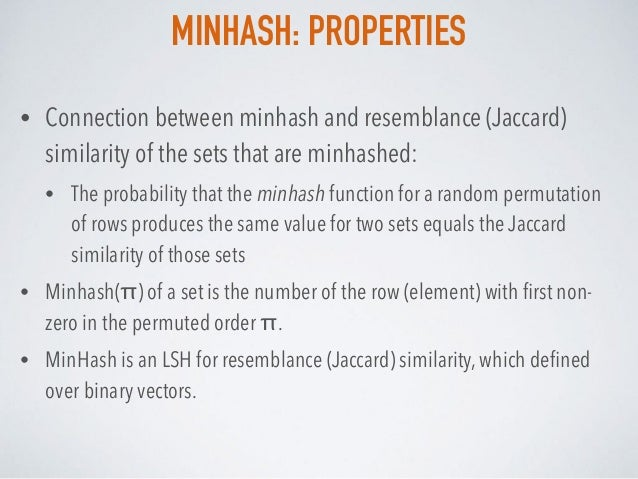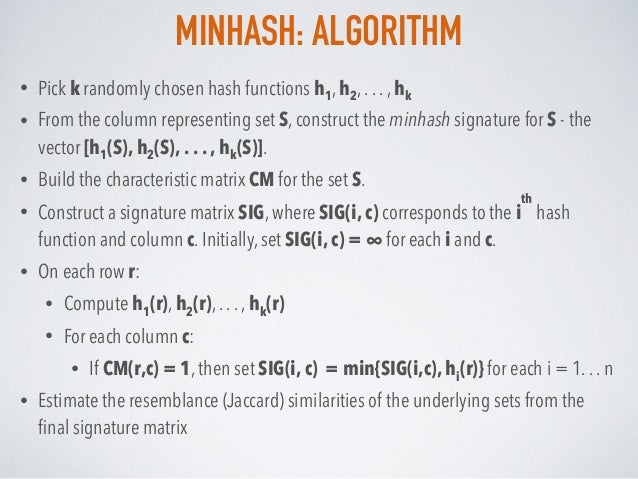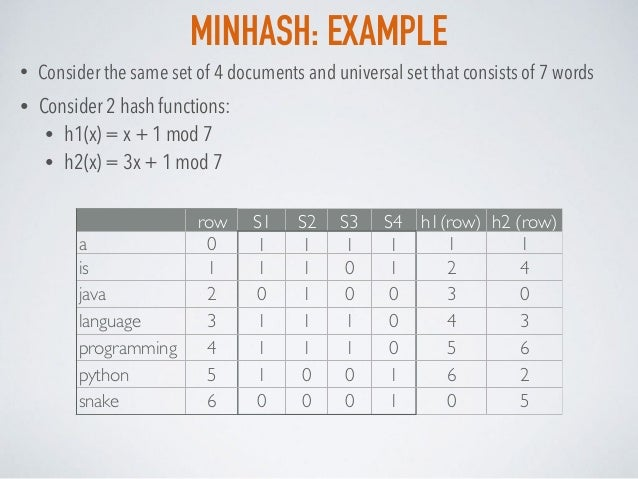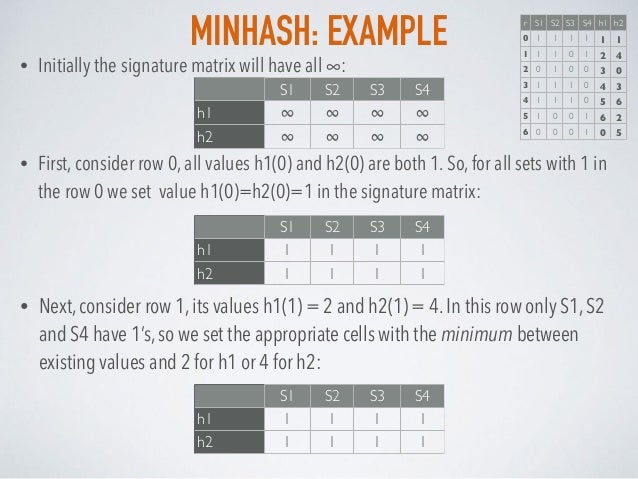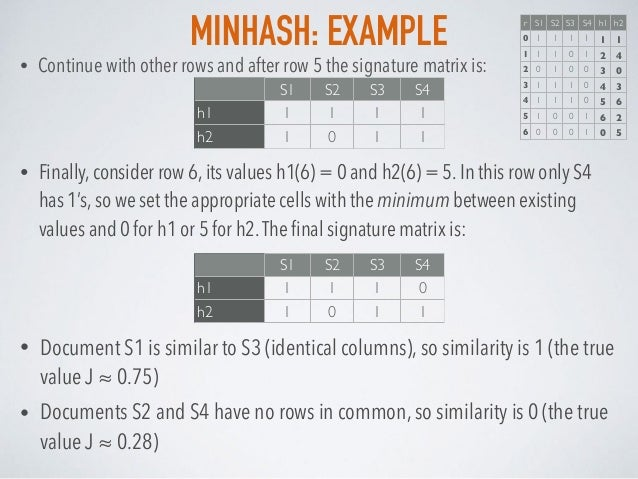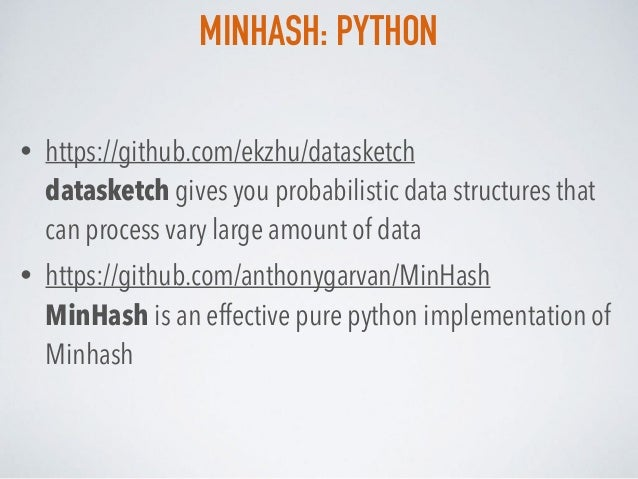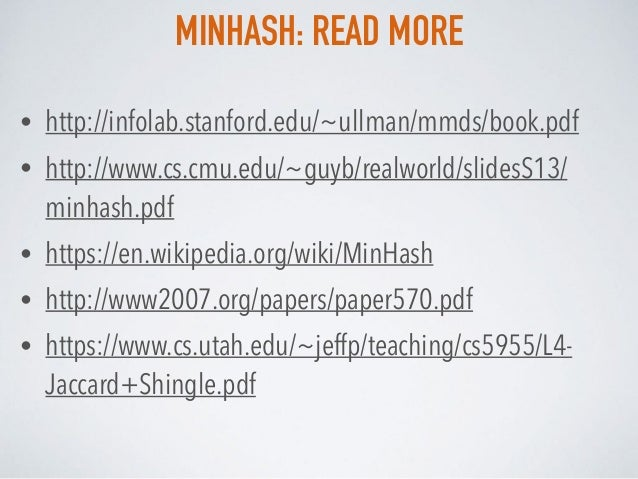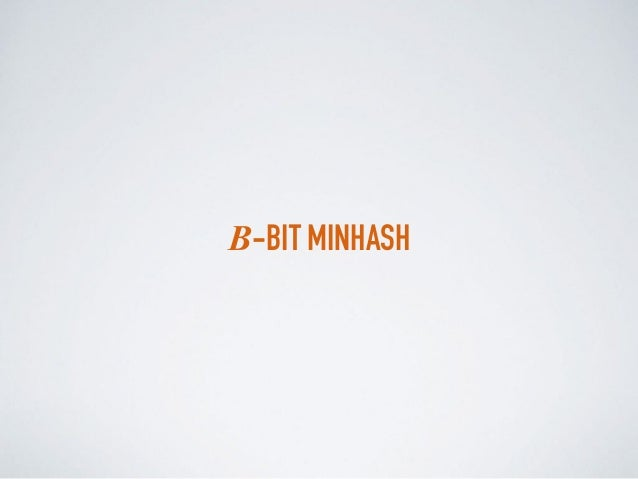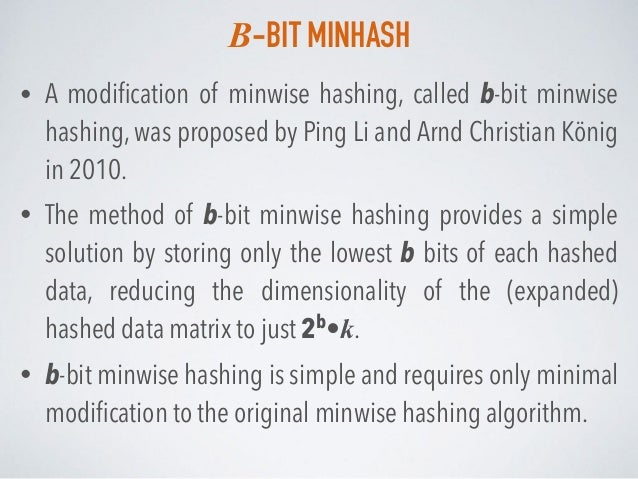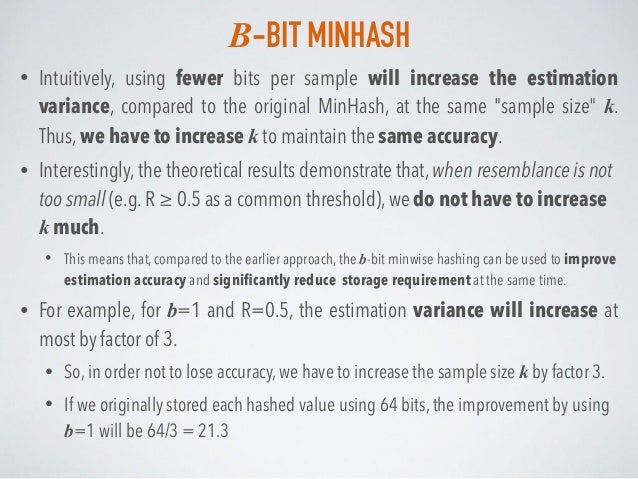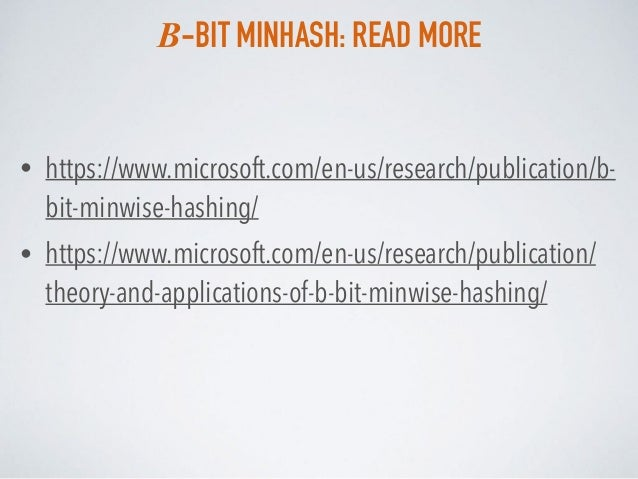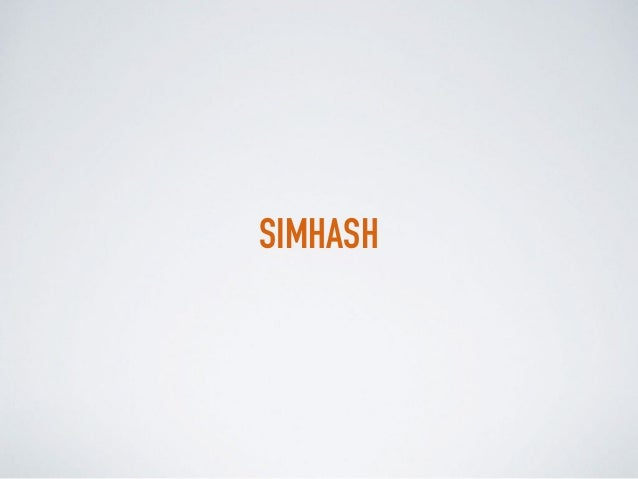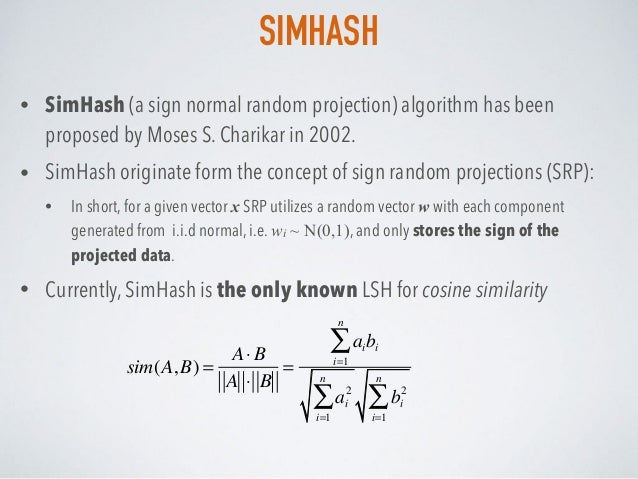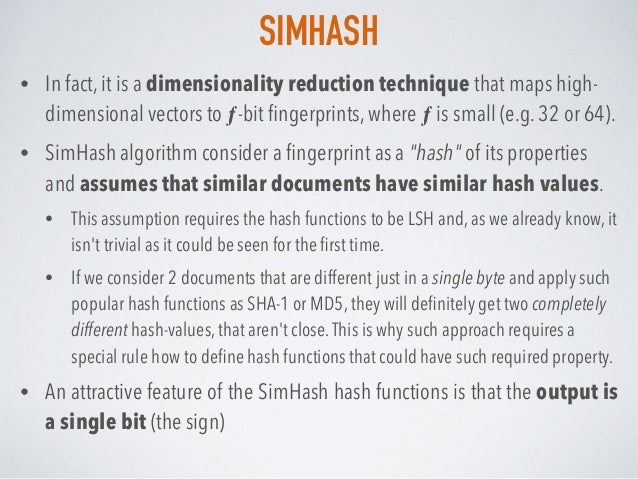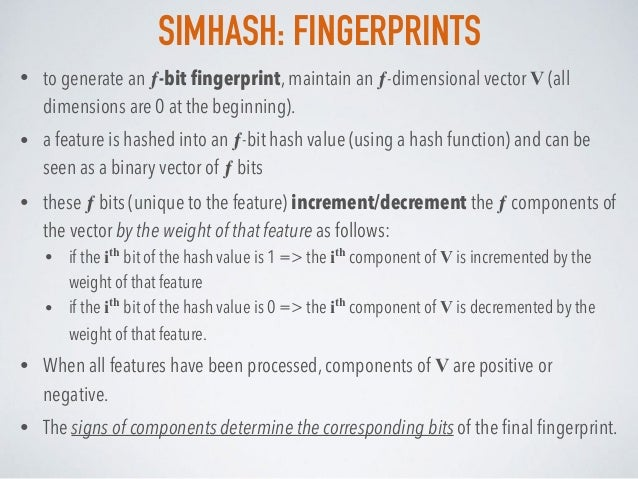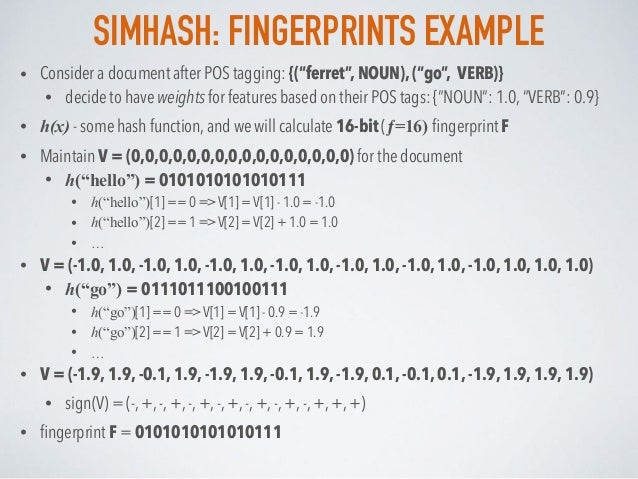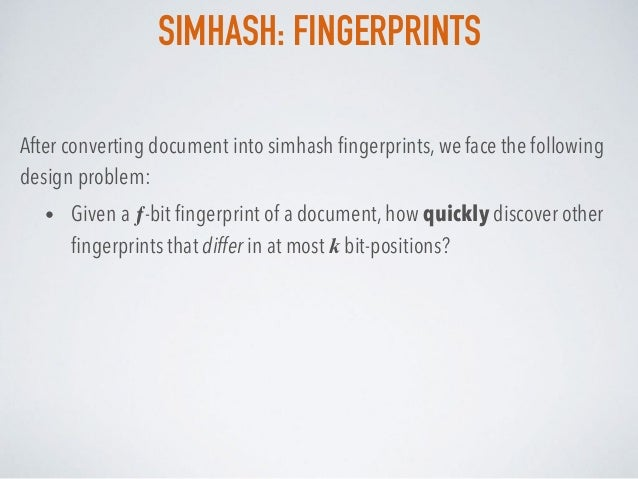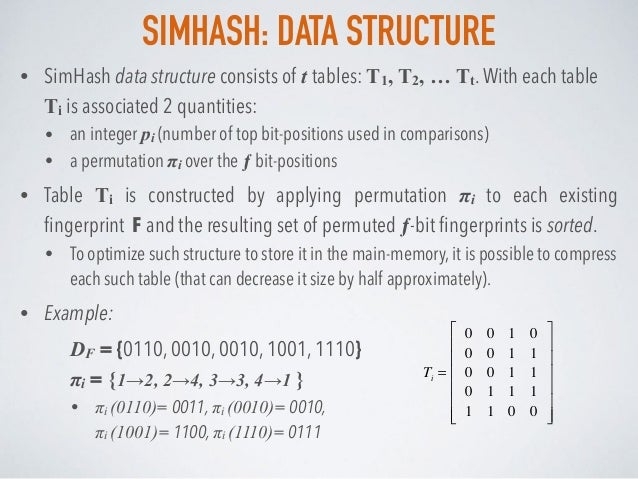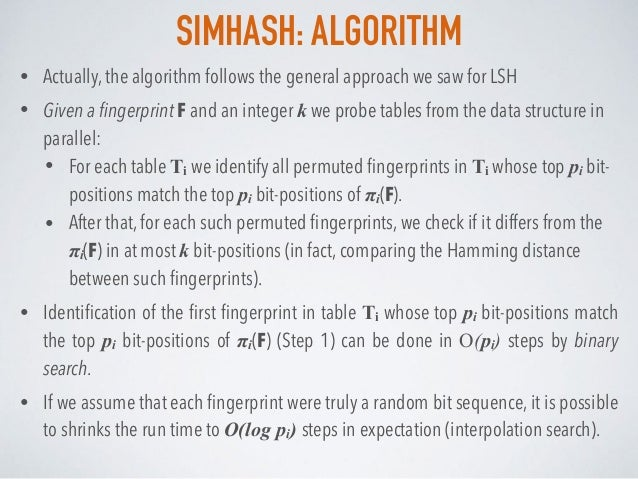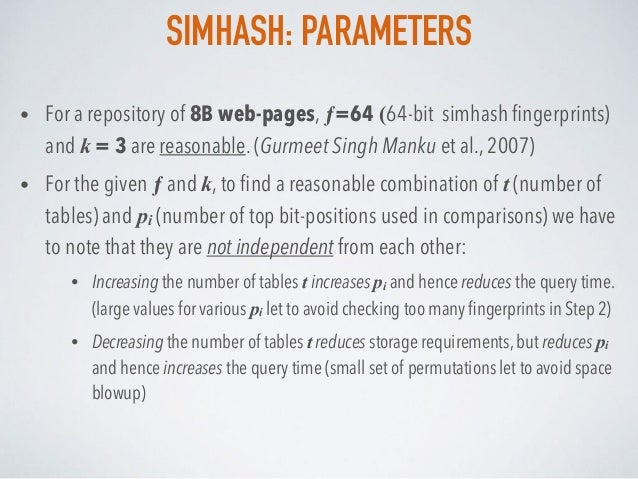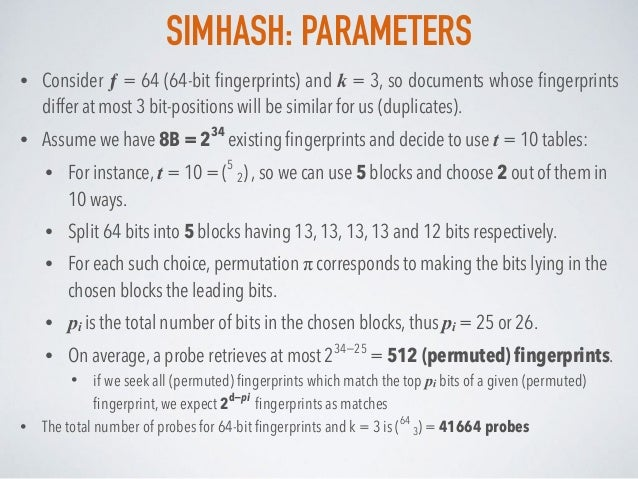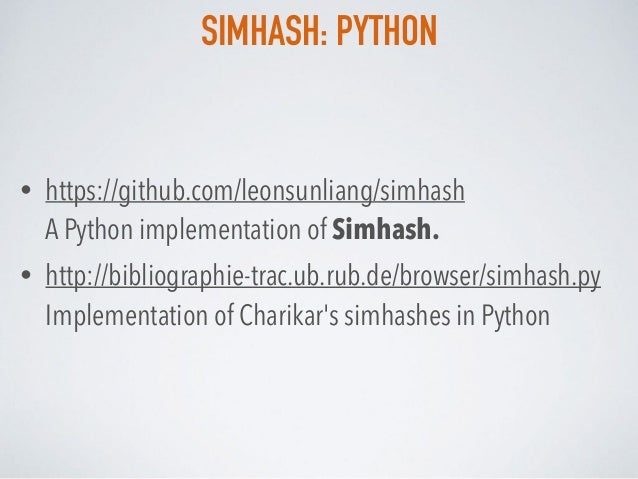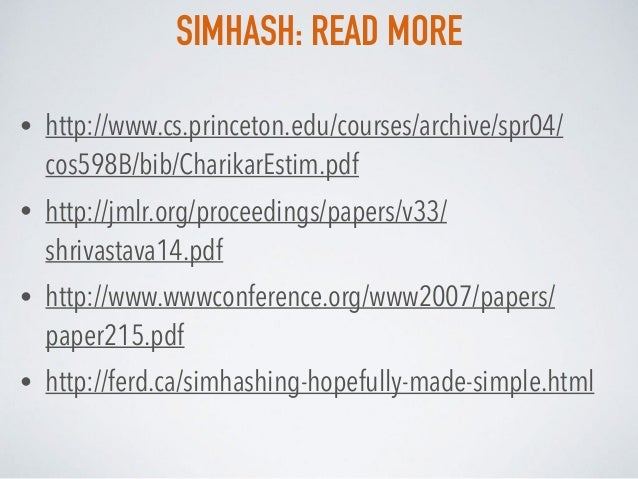Upcoming SlideShare
×
• Full Name
Comment goes here.

Are you sure you want to Yes No• Be the first to comment

### Probabilistic data structures. Part 4. Similarity

1. 1. tech talk @ ferret Andrii Gakhov PROBABILISTIC DATA STRUCTURES ALL YOU WANTED TO KNOW BUT WERE AFRAID TO ASK PART 4: SIMILARITY
2. 2. SIMILARITY Agenda: ▸ Locality-Sensitive Hashing (LSH) ▸ MinHash ▸ SimHash
3. 3. • To ﬁnd clusters of similar documents from the document set • To ﬁnd duplicates of the document in the document set THE PROBLEM
4. 4. LOCALITY SENSITIVE HASHING
5. 5. LOCALITY SENTSITIVE HASHING • Locality Sentsitive Hashing (LSH) was introduced by Indyk and Motwani in 1998 as a family of functions with the following property: • similar input objects (from the domain of such functions) have a higher probability of colliding in the range space than non-similar ones • LSH differs from conventional and cryptographic hash functions because it aims to maximize the probability of a “collision” for similar items. • Intuitively, LSH is based on the simple idea that, if two points are close together, then after a “projection” operation these two points will remain close together.
6. 6. LSH: PROPERTIES • One general approach to LSH is to “hash” items several times, in such a way that similar items are more likely to be hashed to the same bucket than dissimilar items are. We then consider any pair that hashed to the same bucket for any of the hashings to be a candidate pair.
7. 7. LSH: PROPERTIES • Examples of LSH functions are known for resemblance, cosine and hamming distances. • LSH functions do not exist for certain commonly used similarity measures in information retrieval, the Dice coefﬁcient and the Overlap coefﬁcient (Moses S. Charikar, 2002)
8. 8. LSH: PROBLEM • Given a query point, we wish to ﬁnd the points in a large database that are closest to the query. We wish to guarantee with a high probability equal to 1 − δ that we return the nearest neighbor for any query point. • Conceptually, this problem is easily solved by iterating through each point in the database and calculating the distance to the query object. However, our database may contain billions of objects — each object described by a vector that contains hundreds of dimensions. • LSH proves useful to identify nearest neighbors quickly even when the database is very large.
9. 9. LSH: FINDING DUPLICATE PAGES ON THE WEB How to select features for the webpage? • document vector from page content • Compute “document-vector” by case-folding, stop-word removal, stemming, computing term-frequencies and ﬁnally, weighting each term by its inverse document frequency (IDF) • shingles from page content • Consider a document as an sequence of words.A shingle is a hash value of a k-gram which is sub-sequence of k consequent words. • Hashes for shingles can be computed using Rabin’s ﬁngerprints • connectivity information • The idea that similar web pages have several incoming links in common. • anchor text and anchor window • The idea that similar documents should have similar anchor text.The words in the anchor text or anchor window are folded into the document-vector itself. • phrases • Idea is to identify phrases and compute a document-vector that includes phrases as terms.
10. 10. LSH: FINDING DUPLICATE PAGES ON THE WEB • The Web contains many duplicate pages, partly because content is duplicated across sites and partly because there is more than one URL that points to the same page. • If we use shingles as features, each shingle represents a portion of a Web page and is computed by forming a histogram of the words found within that portion of the page. • We can test to see if a portion of the page is duplicated elsewhere on the Web by looking for other shingles with the same histogram. If the shingles of the new page match shingles from the database, then it is likely that the new page bears a strong resemblance to an existing page. • Because Web pages are surrounded by navigational and other information that changes from site to site it is useful to use nearest-neighbor solution that can employ LSH functions.
11. 11. LSH: RETRIEVING IMAGES • LSH can be used in image retrieval as an object recognition tool. We compute a detailed metric for many different orientations and conﬁgurations of an object we wish to recognize. • Then, given a new image we simply check our database to see if a precomputed object’s metrics are close to our query. This database contains millions of poses and LSH allows us to quickly check if the query object is known.
12. 12. LSH: RETRIEVING MUSIC • In music retrieval conventional hashes and robust features are typically used to ﬁnd musical matches. • The features can be ﬁngerprints, i.e., representations of the audio signal that are robust to common types of abuse that are performed to audio before it reaches our ears. • Fingerprints can be computed, for instance, by noting the peaks in the spectrum (because they are robust to noise) and encoding their position in time and space. User then just has to query the database for the same ﬁngerprint. • However, to ﬁnd similar songs we cannot use ﬁngerprints because these are different when a song is remixed for a new audience or when a different artist performs the same song. Instead, we can use several seconds of the song— a snippet—as a shingle. • To determine if two songs are similar, we need to query the database and see if a large enough number of the query shingles are close to one song in the database. • Although closeness depends on the feature vector, we know that long shingles provide speciﬁcity. This is particularly important because we can eliminate duplicates to improve search results and to link recommendation data between similar songs.
13. 13. LSH: PYTHON • https://github.com/ekzhu/datasketch  datasketch gives you probabilistic data structures that can process vary large amount of data • https://github.com/simonemainardi/LSHash  LSHash is a fast Python implementation of locality sensitive hashing with persistence support • https://github.com/go2starr/lshhdc  LSHHDC: Locality-Sensitive Hashing based High Dimensional Clustering
14. 14. LSH: READ MORE • http://www.cs.princeton.edu/courses/archive/spr04/cos598B/ bib/IndykM-curse.pdf • http://infolab.stanford.edu/~ullman/mmds/book.pdf • http://www.cs.princeton.edu/courses/archive/spr04/cos598B/ bib/CharikarEstim.pdf • http://www.matlabi.ir/wp-content/uploads/bank_papers/ g_paper/g15_Matlabi.ir_Locality- Sensitive%20Hashing%20for%20Finding%20Nearest%20Nei ghbors.pdf • https://en.wikipedia.org/wiki/Locality-sensitive_hashing
15. 15. MINHASH
16. 16. MINHASH: RESEMBLANCE SIMILARITY • While talking about relations of two documents A and B, we mostly interesting in such concept as "roughly the same", that could be mathematically formalized as their resemblance (or Jaccard similarity). • Every document can be seen as a set of features and such issue could be reduced to a set intersection problem (ﬁnd similarity between sets). • The resemblance r(A,B) of two documents is a number between 0 and 1, such that it is close to 1 for the documents that are "roughly the same”: • For duplicate detection problem we can reformulate is as a task to ﬁnd pairs of documents whose resemblance r(A,B) exceeds certain threshold R. • For the clustering problem we can use d(A,B) = 1 - r(A,B) as a metric to design algorithms intended to cluster a collection of documents into sets of closely resembling documents. J(A,B) = r(A,B) = A∩ B A∪ B
17. 17. MINHASH • MinHash (minwise hashing algorithm) has been proposed by Andrei Broder in 1997 • The minwise hashing family applies a random permutation π: Ω → Ω, on the given set S, and stores only the minimum values after the permutation mapping. • Formally MinHash is deﬁned as: hπ min S( )= min π S( )( )= min π1 S( ),…πk S( )( )
18. 18. MINHASH: MATRIX REPRESENTATION • Collection of sets could be visualised as characteristic matrix CM. • The columns of the matrix correspond to the sets, and the rows correspond to elements of the universal set from which elements of the sets are drawn. • CM(r, c) =1 if the element for row r is a member of the set for column c, otherwise CM(r,c) =0 • NOTE: The characteristic matrix is unlikely to be the way the data is stored, but it is useful as a way to visualize the data. • For one reason not to store data as a matrix, these matrices are almost always sparse (they have many more 0’s than 1’s) in practice.
19. 19. MINHASH: MATRIX REPRESENTATION • Consider the following sets documents (represented as bag of words): • S1 = {python, is, a, programming, language} • S2 = {java, is, a, programming, language} • S3 = {a, programming, language} • S4 = {python, is, a, snake} • We can assume that our universal set limits to words from these 4 documents.The characteristic matrix of these sets is row S1 S2 S3 S4 a 0 1 1 1 1 is 1 1 1 0 1 java 2 0 1 0 0 language 3 1 1 1 0 programming 4 1 1 1 0 python 5 1 0 0 1 snake 6 0 0 0 1
20. 20. MINHASH: INTUITION • The minhash value of any column of the characteristic matrix is the number of the ﬁrst row, in the permuted order, in which the column has a 1. • To minhash a set represented by such columns, we need to pick a permutation of the rows.
21. 21. MINHASH: ONE STEP EXAMPLE row S1 S2 S3 S4 permuted row 1 1 0 1 0 2 2 1 0 0 0 5 3 0 0 1 1 6 4 1 0 0 1 1 5 0 0 1 1 4 6 0 1 1 0 3 • Consider the characteristic matrix: • Record the ﬁrst “1” in each column: m(S1) = 2, m(S2) = 3, m(S3) = 2, m(S4) = 6 • Estimate the Jaccard similarity: J(Si,Sj) = 1 if m(Si) = m(Sj), or 0 otherwise J(S1,S3) = 1, J(S1, S4) = 0
22. 22. MINHASH: INTUITION • It is not feasible to permute a large characteristic matrix explicitly. • Even picking a random permutation of millions of rows is time- consuming, and the necessary sorting of the rows would take even more time. • Fortunately, it is possible to simulate the effect of a random permutation by a random hash function that maps row numbers to as many buckets as there are rows. • So, instead of picking n random permutations of rows, we pick n randomly chosen hash functions h1, h2, . . . , hn on the rows
23. 23. MINHASH: PROPERTIES • Connection between minhash and resemblance (Jaccard) similarity of the sets that are minhashed: • The probability that the minhash function for a random permutation of rows produces the same value for two sets equals the Jaccard similarity of those sets • Minhash(π) of a set is the number of the row (element) with ﬁrst non- zero in the permuted order π. • MinHash is an LSH for resemblance (Jaccard) similarity, which deﬁned over binary vectors.
24. 24. MINHASH: ALGORITHM • Pick k randomly chosen hash functions h1, h2, . . . , hk • From the column representing set S, construct the minhash signature for S - the vector [h1(S), h2(S), . . . , hk(S)]. • Build the characteristic matrix CM for the set S. • Construct a signature matrix SIG, where SIG(i, c) corresponds to the i th hash function and column c. Initially, set SIG(i, c) = ∞ for each i and c. • On each row r: • Compute h1(r), h2(r), . . . , hk(r) • For each column c: • If CM(r,c) = 1, then set SIG(i, c) = min{SIG(i,c), hi(r)} for each i = 1. . . n • Estimate the resemblance (Jaccard) similarities of the underlying sets from the ﬁnal signature matrix
25. 25. MINHASH: EXAMPLE • Consider 2 hash functions: • h1(x) = x + 1 mod 7 • h2(x) = 3x + 1 mod 7 row S1 S2 S3 S4 h1(row) h2 (row) a 0 1 1 1 1 1 1 is 1 1 1 0 1 2 4 java 2 0 1 0 0 3 0 language 3 1 1 1 0 4 3 programming 4 1 1 1 0 5 6 python 5 1 0 0 1 6 2 snake 6 0 0 0 1 0 5 • Consider the same set of 4 documents and universal set that consists of 7 words
26. 26. MINHASH: EXAMPLE S1 S2 S3 S4 h1 ∞ ∞ ∞ ∞ h2 ∞ ∞ ∞ ∞ • Initially the signature matrix will have all ∞: • First, consider row 0, all values h1(0) and h2(0) are both 1. So, for all sets with 1 in the row 0 we set value h1(0)=h2(0)=1 in the signature matrix: r S1 S2 S3 S4 h1 h2 0 1 1 1 1 1 1 1 1 1 0 1 2 4 2 0 1 0 0 3 0 3 1 1 1 0 4 3 4 1 1 1 0 5 6 5 1 0 0 1 6 2 6 0 0 0 1 0 5 S1 S2 S3 S4 h1 1 1 1 1 h2 1 1 1 1 • Next, consider row 1, its values h1(1) = 2 and h2(1) = 4. In this row only S1, S2 and S4 have 1’s, so we set the appropriate cells with the minimum between existing values and 2 for h1 or 4 for h2: S1 S2 S3 S4 h1 1 1 1 1 h2 1 1 1 1
27. 27. MINHASH: EXAMPLE S1 S2 S3 S4 h1 1 1 1 1 h2 1 0 1 1 • Continue with other rows and after row 5 the signature matrix is: • Finally, consider row 6, its values h1(6) = 0 and h2(6) = 5. In this row only S4 has 1’s, so we set the appropriate cells with the minimum between existing values and 0 for h1 or 5 for h2.The ﬁnal signature matrix is: S1 S2 S3 S4 h1 1 1 1 0 h2 1 0 1 1 r S1 S2 S3 S4 h1 h2 0 1 1 1 1 1 1 1 1 1 0 1 2 4 2 0 1 0 0 3 0 3 1 1 1 0 4 3 4 1 1 1 0 5 6 5 1 0 0 1 6 2 6 0 0 0 1 0 5 • Document S1 is similar to S3 (identical columns), so similarity is 1 (the true value J ≈ 0.75) • Documents S2 and S4 have no rows in common, so similarity is 0 (the true value J ≈ 0.28)
28. 28. MINHASH: PYTHON • https://github.com/ekzhu/datasketch  datasketch gives you probabilistic data structures that can process vary large amount of data • https://github.com/anthonygarvan/MinHash  MinHash is an effective pure python implementation of Minhash
29. 29. MINHASH: READ MORE • http://infolab.stanford.edu/~ullman/mmds/book.pdf • http://www.cs.cmu.edu/~guyb/realworld/slidesS13/ minhash.pdf • https://en.wikipedia.org/wiki/MinHash • http://www2007.org/papers/paper570.pdf • https://www.cs.utah.edu/~jeffp/teaching/cs5955/L4- Jaccard+Shingle.pdf
30. 30. B-BIT MINHASH
31. 31. B-BIT MINHASH • A modiﬁcation of minwise hashing, called b-bit minwise hashing, was proposed by Ping Li and Arnd Christian König in 2010. • The method of b-bit minwise hashing provides a simple solution by storing only the lowest b bits of each hashed data, reducing the dimensionality of the (expanded) hashed data matrix to just 2b •k. • b-bit minwise hashing is simple and requires only minimal modiﬁcation to the original minwise hashing algorithm.
32. 32. B-BIT MINHASH • Intuitively, using fewer bits per sample will increase the estimation variance, compared to the original MinHash, at the same "sample size" k. Thus, we have to increase k to maintain the same accuracy. • Interestingly, the theoretical results demonstrate that, when resemblance is not too small (e.g. R ≥ 0.5 as a common threshold), we do not have to increase k much. • This means that, compared to the earlier approach, the b-bit minwise hashing can be used to improve estimation accuracy and signiﬁcantly reduce storage requirement at the same time. • For example, for b=1 and R=0.5, the estimation variance will increase at most by factor of 3. • So, in order not to lose accuracy, we have to increase the sample size k by factor 3. • If we originally stored each hashed value using 64 bits, the improvement by using b=1 will be 64/3 = 21.3
33. 33. B-BIT MINHASH: READ MORE • https://www.microsoft.com/en-us/research/publication/b- bit-minwise-hashing/ • https://www.microsoft.com/en-us/research/publication/ theory-and-applications-of-b-bit-minwise-hashing/
34. 34. SIMHASH
35. 35. SIMHASH • SimHash (a sign normal random projection) algorithm has been proposed by Moses S. Charikar in 2002. • SimHash originate form the concept of sign random projections (SRP): • In short, for a given vector x SRP utilizes a random vector w with each component generated from i.i.d normal, i.e. wi ~ N(0,1), and only stores the sign of the projected data. • Currently, SimHash is the only known LSH for cosine similarity sim(A,B) = A⋅ B A ⋅ B = aibi i=1 n ∑ ai 2 i=1 n ∑ bi 2 i=1 n ∑
36. 36. SIMHASH • In fact, it is a dimensionality reduction technique that maps high- dimensional vectors to ƒ-bit ﬁngerprints, where ƒ is small (e.g. 32 or 64). • SimHash algorithm consider a ﬁngerprint as a "hash" of its properties and assumes that similar documents have similar hash values. • This assumption requires the hash functions to be LSH and, as we already know, it isn't trivial as it could be seen for the ﬁrst time. • If we consider 2 documents that are different just in a single byte and apply such popular hash functions as SHA-1 or MD5, they will deﬁnitely get two completely different hash-values, that aren't close.This is why such approach requires a special rule how to deﬁne hash functions that could have such required property. • An attractive feature of the SimHash hash functions is that the output is a single bit (the sign)
37. 37. SIMHASH: FINGERPRINTS • to generate an ƒ-bit ﬁngerprint, maintain an ƒ-dimensional vector V (all dimensions are 0 at the beginning). • a feature is hashed into an ƒ-bit hash value (using a hash function) and can be seen as a binary vector of ƒ bits • these ƒ bits (unique to the feature) increment/decrement the ƒ components of the vector by the weight of that feature as follows: • if the ith bit of the hash value is 1 => the ith component of V is incremented by the weight of that feature • if the ith bit of the hash value is 0 => the ith component of V is decremented by the weight of that feature. • When all features have been processed, components of V are positive or negative. • The signs of components determine the corresponding bits of the ﬁnal ﬁngerprint.
38. 38. SIMHASH: FINGERPRINTS EXAMPLE • Consider a document after POS tagging: {(“ferret”, NOUN), (“go”, VERB)} • decide to have weights for features based on their POS tags: {“NOUN”: 1.0, “VERB”: 0.9} • h(x) - some hash function, and we will calculate 16-bit (ƒ=16) ﬁngerprint F • Maintain V = (0,0,0,0,0,0,0,0,0,0,0,0,0,0,0,0) for the document • h(“hello”) = 0101010101010111 • h(“hello”) == 0 => V = V - 1.0 = -1.0 • h(“hello”) == 1 => V = V + 1.0 = 1.0 • … • V = (-1.0, 1.0, -1.0, 1.0, -1.0, 1.0, -1.0, 1.0, -1.0, 1.0, -1.0, 1.0, -1.0, 1.0, 1.0, 1.0) • h(“go”) = 0111011100100111 • h(“go”) == 0 => V = V - 0.9 = -1.9 • h(“go”) == 1 => V = V + 0.9 = 1.9 • … • V = (-1.9, 1.9, -0.1, 1.9, -1.9, 1.9, -0.1, 1.9, -1.9, 0.1, -0.1, 0.1, -1.9, 1.9, 1.9, 1.9) • sign(V) = (-, +, -, +, -, +, -, +, -, +, -, +, -, +, +, +) • ﬁngerprint F = 0101010101010111
39. 39. SIMHASH: FINGERPRINTS After converting document into simhash ﬁngerprints, we face the following design problem: • Given a ƒ-bit ﬁngerprint of a document, how quickly discover other ﬁngerprints that differ in at most k bit-positions?
40. 40. SIMHASH: DATA STRUCTURE • SimHash data structure consists of t tables: T1, T2, … Tt.With each table Ti is associated 2 quantities: • an integer pi (number of top bit-positions used in comparisons) • a permutation πi over the ƒ bit-positions • Table Ti is constructed by applying permutation πi to each existing ﬁngerprint F and the resulting set of permuted ƒ-bit ﬁngerprints is sorted. • To optimize such structure to store it in the main-memory, it is possible to compress each such table (that can decrease it size by half approximately). • Example: DF = {0110, 0010, 0010, 1001, 1110}  πi = {1→2, 2→4, 3→3, 4→1 } • πi (0110)= 0011, πi (0010)= 0010,  πi (1001)= 1100, πi (1110)= 0111 Ti = 0 0 1 0 0 0 1 1 0 0 1 1 0 1 1 1 1 1 0 0 ⎡ ⎣ ⎢ ⎢ ⎢ ⎢ ⎢ ⎢ ⎤ ⎦ ⎥ ⎥ ⎥ ⎥ ⎥ ⎥
41. 41. SIMHASH: ALGORITHM • Actually, the algorithm follows the general approach we saw for LSH • Given a ﬁngerprint F and an integer k we probe tables from the data structure in parallel: • For each table Ti we identify all permuted ﬁngerprints in Ti whose top pi bit- positions match the top pi bit-positions of πi(F). • After that, for each such permuted ﬁngerprints, we check if it differs from the πi(F) in at most k bit-positions (in fact, comparing the Hamming distance between such ﬁngerprints). • Identiﬁcation of the ﬁrst ﬁngerprint in table Ti whose top pi bit-positions match the top pi bit-positions of πi(F) (Step 1) can be done in O(pi) steps by binary search. • If we assume that each ﬁngerprint were truly a random bit sequence, it is possible to shrinks the run time to O(log pi) steps in expectation (interpolation search).
42. 42. SIMHASH: PARAMETERS • For a repository of 8B web-pages, ƒ=64 (64-bit simhash ﬁngerprints) and k = 3 are reasonable. (Gurmeet Singh Manku et al., 2007) • For the given ƒ and k, to ﬁnd a reasonable combination of t (number of tables) and pi (number of top bit-positions used in comparisons) we have to note that they are not independent from each other: • Increasing the number of tables t increases pi and hence reduces the query time. (large values for various pi let to avoid checking too many ﬁngerprints in Step 2) • Decreasing the number of tables t reduces storage requirements, but reduces pi and hence increases the query time (small set of permutations let to avoid space blowup)
43. 43. SIMHASH: PARAMETERS • Consider ƒ = 64 (64-bit ﬁngerprints) and k = 3, so documents whose ﬁngerprints differ at most 3 bit-positions will be similar for us (duplicates). • Assume we have 8B = 234 existing ﬁngerprints and decide to use t = 10 tables: • For instance, t = 10 = (5 2) , so we can use 5 blocks and choose 2 out of them in 10 ways. • Split 64 bits into 5 blocks having 13, 13, 13, 13 and 12 bits respectively. • For each such choice, permutation π corresponds to making the bits lying in the chosen blocks the leading bits. • pi is the total number of bits in the chosen blocks, thus pi = 25 or 26. • On average, a probe retrieves at most 234−25 = 512 (permuted) ﬁngerprints. • if we seek all (permuted) ﬁngerprints which match the top pi bits of a given (permuted) ﬁngerprint, we expect 2d−pi ﬁngerprints as matches • The total number of probes for 64-bit ﬁngerprints and k = 3 is (64 3) = 41664 probes
44. 44. SIMHASH: PYTHON • https://github.com/leonsunliang/simhash  A Python implementation of Simhash. • http://bibliographie-trac.ub.rub.de/browser/simhash.py  Implementation of Charikar's simhashes in Python
45. 45. SIMHASH: READ MORE • http://www.cs.princeton.edu/courses/archive/spr04/ cos598B/bib/CharikarEstim.pdf • http://jmlr.org/proceedings/papers/v33/ shrivastava14.pdf • http://www.wwwconference.org/www2007/papers/ paper215.pdf • http://ferd.ca/simhashing-hopefully-made-simple.html
46. 46. ▸ @gakhov ▸ linkedin.com/in/gakhov ▸ www.datacrucis.com THANK YOU

### Be the first to comment

•#### shalinivbatar

Jun. 5, 2017
•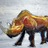#### mring33

Sep. 28, 2017
•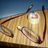#### tellzeta

Aug. 29, 2019

Total views

2,102

On Slideshare

0

From embeds

0

Number of embeds

150

69

Shares

0